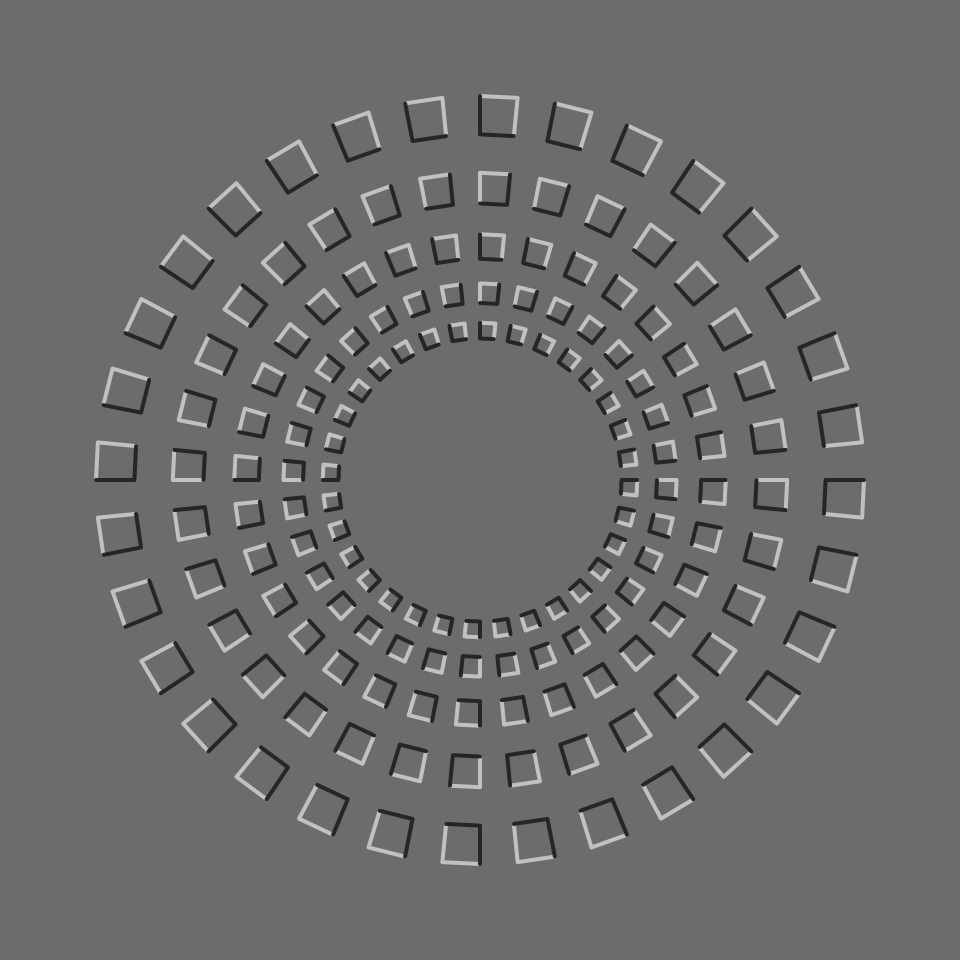The Pinna illusion http://www.scholarpedia.org/article/Pinna_illusion

library(grid)
grid.newpage()
grid.rect(gp=gpar(col=NA, fill="grey50"))
N = 32; R0 = 0.8; Rf = 0.8; NR = 5
lwd = 2
cols = c("gray20", "gray80")
for(j in 1:NR) {
r = R0*Rf^(j-1)
th = seq(0, 2*pi, len = (N*2+1))[-c(1)]
if (j%%2==1) th = rev(th)
px1 = cos(th)*r
px2 = c(matrix(cos(th)*(r*0.90), 2)[2:1,])
py1 = sin(th)*r
py2 = c(matrix(sin(th)*(r*0.90), 2)[2:1,])
grid.polyline(0.5*c(px1, px2[seq(1, length(px2), by=2)])+0.5,
0.5*c(py1, py2[seq(1, length(py2), by=2)])+0.5,
id = c(rep(1:(N), each=2), 1:(N)),
gp = gpar(col = cols, lwd = lwd))
grid.polyline(0.5*c(px2, px1[seq(1, length(px2), by=2)])+0.5,
0.5*c(py2, py1[seq(1, length(py2), by=2)])+0.5,
id = c(rep(1:(N), each=2), 1:(N)),
gp = gpar(col = cols, lwd = lwd))
}